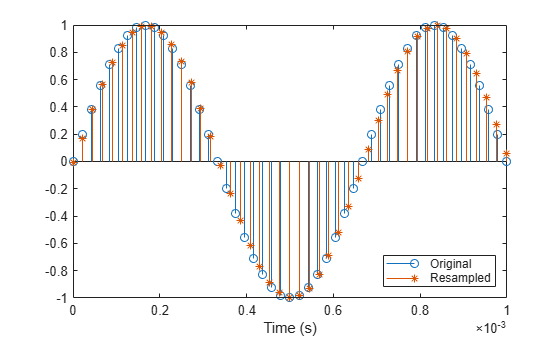Documentation

# upfirdn

Upsample, apply FIR filter, and downsample

## Syntax

``yout = upfirdn(xin,h)``
``yout = upfirdn(xin,h,p)``
``yout = upfirdn(xin,h,p,q)``

## Description

````yout = upfirdn(xin,h)` filters the input signal `xin` with an FIR filter with impulse response `h`. No upsampling or downsampling is implemented with this syntax.```
````yout = upfirdn(xin,h,p)` specifies the integer upsampling factor `p`.```

example

````yout = upfirdn(xin,h,p,q)` specifies the integer downsampling factor `q`.```

## Examples

collapse all

Change the sample rate of a signal by a rational conversion factor from the DAT rate of 48 kHz to the CD sample rate of 44.1 kHz. Use the `rat` function to find the numerator `L` and the denominator `M` of the rational factor.

```Fdat = 48e3; Fcd = 44.1e3; [L,M] = rat(Fcd/Fdat)```
```L = 147 ```
```M = 160 ```

Generate a 1.5 kHz sinusoid sampled at ${\mathit{f}}_{\mathrm{DAT}}$ for 0.25 seconds. Plot the first millisecond of the signal.

```t = 0:1/Fdat:0.25-1/Fdat; x = sin(2*pi*1.5e3*t); stem(t,x) xlim([0 0.001]) hold on```Design an antialiasing lowpass filter using a Kaiser window. Set the filter band edges as 90% and 110% of the cutoff frequency, $\left({\mathit{f}}_{\mathrm{DAT}}/2\right)×\mathrm{min}\left(1/\mathit{L},1/\mathit{M}\right)$. Specify a passband ripple of 5 dB and a stopband attenuation of 40 dB. Set the passband gain to `L`.

```f = (Fdat/2)*min(1/L,1/M); d = designfilt('lowpassfir', ... 'PassbandFrequency',0.9*f,'StopbandFrequency',1.1*f, ... 'PassbandRipple',5,'StopbandAttenuation',40, ... 'DesignMethod','kaiserwin','SampleRate',48e3); h = L*tf(d);```

Use `upfirdn` with the filter `h` to resample the sinusoid. Compute and compensate for the delay introduced by the filter. Generate the corresponding resampled time vector.

```y = upfirdn(x,h,L,M); delay = floor(((filtord(d)-1)/2-(L-1))/L); y = y(delay+1:end); t_res = (0:(length(y)-1))/Fcd;```

Overlay the resampled signal on the plot.

```stem(t_res,y,'*') legend('Original','Resampled','Location','southeast') hold off```## Input Arguments

collapse all

Input signal, specified by a vector or matrix. If `xin` is a vector, then it represents a single signal. If `xin` is a matrix,then each column is filtered independently.

Filter impulse response, specified as a vector or matrix. If `h`is a vector, then it represents one FIR filter. If `h` is a matrix, then each column is a separate FIR impulse response sequence.

Upsampling factor, specified by a positive integer.

Downsampling factor, specified by a positive integer.

## Output Arguments

collapse all

Output signal, specified as a vector or matrix. If `yout` is a vector, then it represents one signal. If `yout` is a matrix, then each column is a separate output. The length of the output, `yout` is `ceil(((length(xin)-1)*p+length(h))/q)`.

### Note

Since `upfirdn` performs convolution and rate changing, the `yout` signals have a different length than `xin`. The number of rows of `yout` is approximately `p/q` times the number of rows of `xin`.

## Tips

The valid combinations of the sizes of `xin` and `h` are:

1. `xin` is a vector and `h` is a vector.

The inputs are one filter and one signal, so the function convolves `xin` with `h`. The output signal `yout` is a row vector if `xin` is a row vector; otherwise, `yout` is a column vector.

2. `xin` is a matrix and `h` is a vector.

The inputs are one filter and many signals, so the function convolves `h` with each column of `xin`. The resulting `yout` is a matrix with the same number of columns as `xin`.

3. `xin` is a vector and `h` is a matrix.

The inputs are multiple filters and one signal, so the function convolves each column of `h` with `xin`. The resulting `yout` is a matrix with the same number of columns as `h`.

4. `xin` is a matrix and `h` is a matrix, both with the same number of columns.

The inputs are multiple filters and multiple signals, so the function convolves corresponding columns of `xin` and `h`. The resulting `yout` is a matrix with the same number of columns as `xin` and `h`.

## Algorithms

`upfirdn` uses a polyphase interpolation structure. The number of multiply-add operations in the polyphase structure is approximately (LhLx – pLx)/q where Lh and Lx are the lengths of h(n) and x(n), respectively.

A more accurate flops count is computed in the program, but the exact count is still approximate. For long signals x(n), the formula is often exact.

`upfirdn` performs a cascade of three operations:

1. Upsample the input data in the matrix `xin` by a factor of the integer `p` (inserting zeros)

2. FIR filter the upsampled signal data with the impulse response sequence given in the vector or matrix `h`

3. Downsample the result by a factor of the integer `q` (throwing away samples)

The FIR filter is usually a lowpass filter, which you must design using another function such as `firpm` or `fir1`.

### Note

The function `resample` performs an FIR design using `firls`, followed by rate changing implemented with `upfirdn`.

 Crochiere, R. E., and Lawrence R. Rabiner. Multirate Digital Signal Processing. Englewood Cliffs, NJ: Prentice-Hall, 1983, pp. 88–91.

 Crochiere, R. E. “A General Program to Perform Sampling Rate Conversion of Data by Rational Ratios.” Programs for Digital Signal Processing (Digital Signal Processing Committee of the IEEE Acoustics, Speech, and Signal Processing Society, eds.). New York: IEEE Press, 1979, Programs 8.2-1–8.2-7.